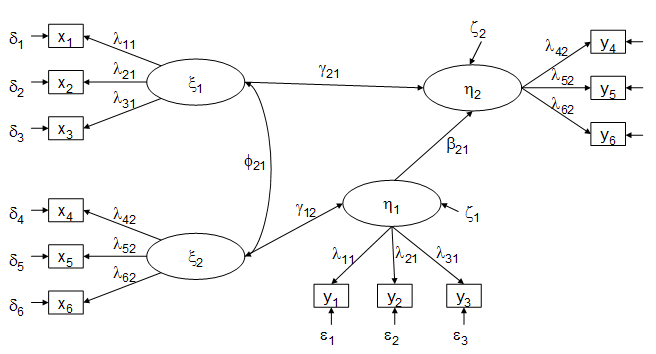## Lecture 1: Overview & Path Analysis#### Topics

Setting the stage: EFA, CFA, SEM, Path analysis

• Goal: Understand relations among a large number of observed variables
• Goal: Extend regression methods to (a) multiple outcomes, (b) latent variables, (c) accounting for measurement error or unreliability
• Thinking: Equations -> Path diagram -> estimate, test, visualize

## Lecture 2: Measurement models & CFA#### Topics

• Effects of measurement error
• Testing equivalence of measures with CFA
• Multi-factor, higher-order models
• Multi-group models: Factorial invariance

• CFA in lavaan. A nice tutorial on fitting CFA models using lavaan. It uses a larger version of the Holzinger-Swineford (1939) data used in the exercise and discusses goodness-of-fit measures, model comparison, and R tools to get nice output for write-ups.

## Lecture 3: SEM with latent variables & other topics#### Topics

• The full SEM model
• Longitudinal data
• Power & sample size
• SEM extensions